# Create a Paint Bucket Tool in HTML5 and JavaScript

This tutorial will show how to create a simple paint bucket tool on an HTML5 Canvas using JavaScript. We will be implementing a flood fill algorithm using the HTML5 imageData object for pixel manipulation.

First we will learn how to determine the colors of the pixel on an HTML5 canvas. Then we will go step by step through an implementation of a flood fill algorithm in JavaScript. Let's get started.

## The HTML5 Canvas Pixel

Before we start filling anything, we need to know the colors of our image. We can utilize HTML5 imageData object to describe our image as an array of pixel data or more specifically a CanvasPixelArray object. Each pixel is in the form: [R, G, B, A] where R is the red component, G is green component, B is the blue component, and A is the alpha.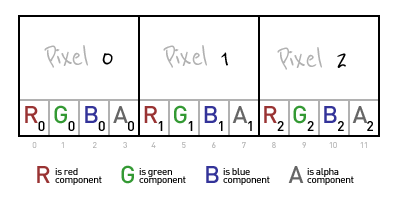The `getImageData` method has four parameters: location (x,y) and dimension (width, height). The JavaScript code looks like this:

`var imgData = context.getImageData(x, y, width, height);`

The image data for an image consisting of one red pixel is described as `[255, 0, 0, 255]`.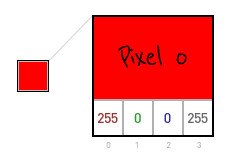```var imgData = context.getImageData(0, 0, 1, 1);

// imageData.data = [255,0,0,255]```

The imageData for a four pixel image (red, black, white, purple) is described as `[255,0,0,255, 0,0,0,255, 255,255,255,255, 203,53,148,255]`.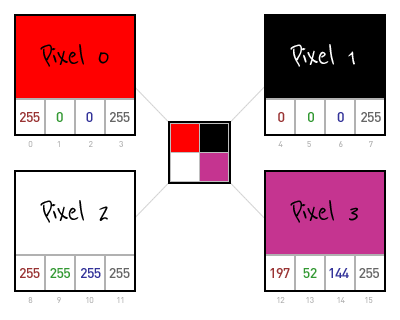```var imgDataArray = context.getImageData(0, 0, 2, 2);

// imageData.data = [255,0,0,255]      /* red    */
// imageData.data = [0,0,0,255]        /* black  */
// imageData.data = [255,255,255,255]  /* white  */
// imageData.data = [203,53,148,255]   /* purple */```

The example image we will use throughout this article is 3 pixels wide and 5 pixels high. It consists of 15 pixels, 13 white `[255,255,255,255]` and 2 black`[0,0,0,255]`. The black pixels are in positions 3 and 7.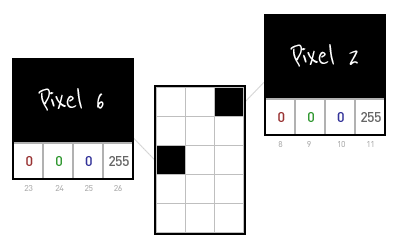```var imageData = context.getImageData(0,0,canvasWidth,canvasHeight);

// imageData.data = [
//   255,255,255,255, 255,255,255,255, 0,0,0,255,
//   255,255,255,255, 255,255,255,255, 255,255,255,255,
//   0,0,0,255,       255,255,255,255, 255,255,255,255,
//   255,255,255,255, 255,255,255,255, 255,255,255,255,
//   255,255,255,255, 255,255,255,255, 255,255,255,255]```

## Preview Flood Fill Animation

Now that we have demonstrated how we will get pixel data from the HTML5 Canvas via the imageData object we will go step by step through the process of a flood fill algorithm implemented with JavaScript.

The following animation shows the steps of the flood fill algorithm on our example image: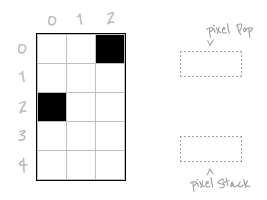## Flood Fill Step by Step

Our objective is to fill of all similarly colored pixels connected to the starting pixel with a new fill color. In our example the starting pixel is white and the fill color is Watermelon Duck purple.

First push the starting pixel's x and y coordinate into an array called `pixelStack`. Let's start at pixel location (1,3).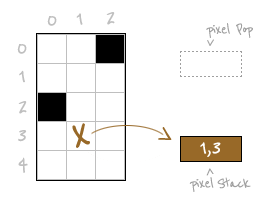`var pixelStack = [[1, 3]];`

Next we create a while loop which will pop the most recently saved pixel location in `pixelStack`. In the case of our example, we pop the only coordinate (1,3) and assign those values to the variables `x` and `y` respectively.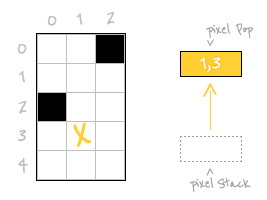```while(pixelStack.length)
{
newPos = pixelStack.pop();
x = newPos;
y = newPos;

...

}```

We travel upward pixel by pixel until we find the image boundary or a pixel that does not match the fill color. We use another while loop and the function `matchStartColor` which returns true if the color matches the starting color.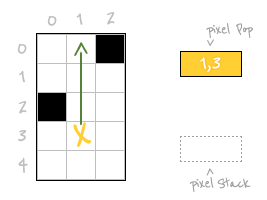```  pixelPos = (y*canvasWidth + x) * 4;
while(y-- >= 0 && matchStartColor(pixelPos))
{
pixelPos -= canvasWidth * 4;
}
...
}

function matchStartColor(pixelPos)
{
var r = colorLayer.data[pixelPos];
var g = colorLayer.data[pixelPos+1];
var b = colorLayer.data[pixelPos+2];

return (r == startR && g == startG && b == startB);
}```

After travelling up 3 pixels we encounter the edge of the image. We know this because `y` becomes -1 the condition `y-- >= 0` fails. Since we have gone too far up, we increment `y` and update the variable `pixelPos`. Instead of just incrementing the pixelPos by one, we increment by the canvas width times 4.

We initalize a couple of new variables `reachLeft` and `reachRight` to false. These will be used to manage additions to our pixel stack. We will see how soon.

We create another while loop, this time travelling downward. Unlike how we went up blindly, the way down will involve adding new pixels to the stack and coloring pixels.

Let's start by coloring our first pixel at (1,0) with the fill color purple.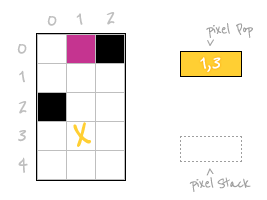```  pixelPos += canvasWidth * 4;
++y;
reachLeft = false;
reachRight = false;
while(y++ < canvasHeight-1 && matchStartColor(pixelPos))
{
colorPixel(pixelPos);

...

}```

After filling the pixel at (1,0) we must now determine if its neighbors to the left and right need filling too. First let's investigate the pixel to the left.

Ignoring any pixels with x position less than zero we check if the color matches the start color. In our example the pixel to the left is white just like our start pixel, so we add it to the stack to handle later. We also set the boolean `reachLeft` to true. This will prevent us adding pixels that will eventually handled by the downward march of the pixel we just added.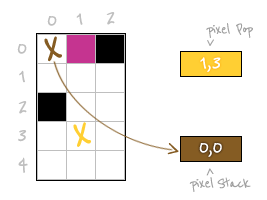```    if(x > 0)
{
if(matchStartColor(pixelPos - 4))
{
if(!reachLeft){
pixelStack.push([x - 1, y]);
reachLeft = true;
}
}
else if(reachLeft)
{
reachLeft = false;
}
}```

Now we look to the right. The pixel on the right is black which does not match the starting pixel so we do not add it to the stack. We continue the march downward.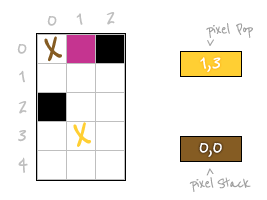```    if(x < canvasWidth-1)
{
if(matchStartColor(pixelPos + 4))
{
if(!reachRight)
{
pixelStack.push([x + 1, y]);
reachRight = true;
}
}
else if(reachRight)
{
reachRight = false;
}
}

pixelPos += canvasWidth * 4;```

The pixel below matches our starting pixel so we color it purple.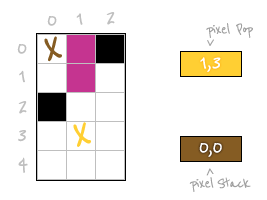We look to the left of our new purple pixel and find it matches, but `reachLeft` is true so we don't add it to the stack. The right pixel also matches but its variable `reachRight` is false so we do add it to the stack.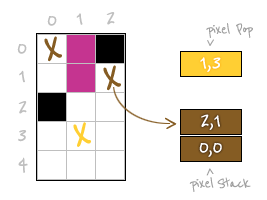Color the next pixel. Look to the left, no match this time so we change `reachLeft` to false. Looking to the right matches but `reachRight` is true so we do nothing.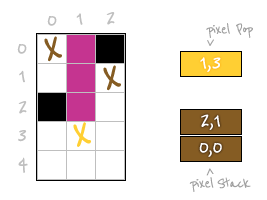Color the next pixel. It happens to be the starting pixel but other than for reasons of nostalgia it's not significant.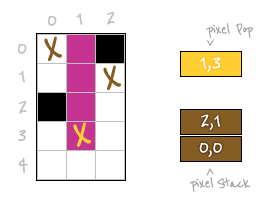We look to the left and the color matches. Since we changed `reachLeft` to false last time, we add it to the stack. The right side matches too, but its boolean `reachRight` is true so we don't add it.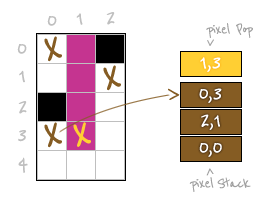Color the pixel below. The booleans `reachLeft` and `reachRight` to the right and left are true so we don't add them to the stack.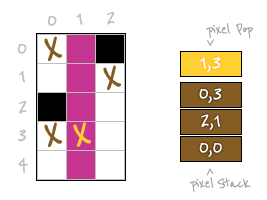We have reached the bottom of the image thus are done with the column of our starting pixel.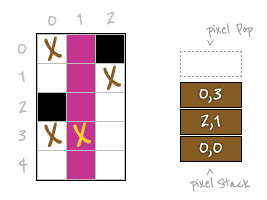## Empty the Stack

The first column has been filled however they are still pixels in the stack which means there are more columns to fill. Pop the next pixel from the stack which is the pixel (0,3).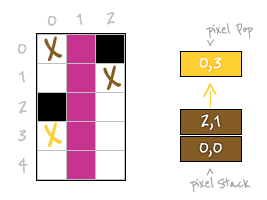We continue the process until the pixel stack is empty and there are no more columns to fill.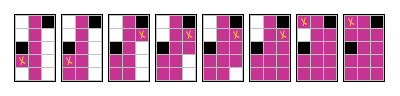Eight pixels later we have filled all the matching pixels in our example image. Although our example image was small the process is the same for larger images.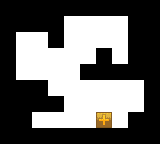## HTML5 JS Paint Bucket Code

A few things to note about the code:

• Internet Explorer does not support HTML5 pixel manipulation even with use of ExplorerCanvas
• Firefox does not support pixel manipulation locally. It will work when hosted on a site, but if you try to test locally you will what Firebug describes as 'Security error" code: "1000'. The best explanation for this error was described by skarabaeus here.```pixelStack = [[startX, startY]];

while(pixelStack.length)
{
var newPos, x, y, pixelPos, reachLeft, reachRight;
newPos = pixelStack.pop();
x = newPos;
y = newPos;

pixelPos = (y*canvasWidth + x) * 4;
while(y-- >= drawingBoundTop && matchStartColor(pixelPos))
{
pixelPos -= canvasWidth * 4;
}
pixelPos += canvasWidth * 4;
++y;
reachLeft = false;
reachRight = false;
while(y++ < canvasHeight-1 && matchStartColor(pixelPos))
{
colorPixel(pixelPos);

if(x > 0)
{
if(matchStartColor(pixelPos - 4))
{
if(!reachLeft){
pixelStack.push([x - 1, y]);
reachLeft = true;
}
}
else if(reachLeft)
{
reachLeft = false;
}
}

if(x < canvasWidth-1)
{
if(matchStartColor(pixelPos + 4))
{
if(!reachRight)
{
pixelStack.push([x + 1, y]);
reachRight = true;
}
}
else if(reachRight)
{
reachRight = false;
}
}

pixelPos += canvasWidth * 4;
}
}
context.putImageData(colorLayer, 0, 0);

function matchStartColor(pixelPos)
{
var r = colorLayer.data[pixelPos];
var g = colorLayer.data[pixelPos+1];
var b = colorLayer.data[pixelPos+2];

return (r == startR && g == startG && b == startB);
}

function colorPixel(pixelPos)
{
colorLayer.data[pixelPos] = fillColorR;
colorLayer.data[pixelPos+1] = fillColorG;
colorLayer.data[pixelPos+2] = fillColorB;
colorLayer.data[pixelPos+3] = 255;
}
```